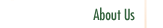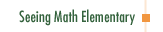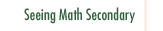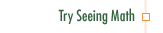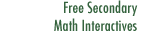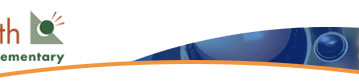Geometry: 2D and 3D Figures

In Geometry: 2D and 3D Figures, students explore the terminology and properties of three-dimensional solids. They visualize solid figures, learn the names of their parts, and build and draw figures in two and three dimensions.

Participants in this course:

• Examine the development of student geometric thought, using the four levels of abstraction defined in the van Hiele framework (visual, descriptive/analytic, abstract/relational, formal deduction and proof).
• Consider ways to support student-initiated inquiry in a classroom.
• Examine questioning strategies that support concept clarification, reflection, and discussion.
• Explore how activities such as making models or writing geometric puzzles can reinforce students' understanding of geometric figures, and provide a way to assess students' geometric thinking.
• Reflect on their own understanding of geometry.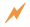Click to Get Seeing Math™ ElementaryCourse Listing Effective Questioning in the Mathematics Classroom Foundations of EffectiveMathematics Teaching Formative Assessment in the Mathematics Classroom Number & Operations: Division with Remainders Number & Operations: The Magnitude of Fractions Number & Operations: Broken Calculator Geometry: 2D and 3D Figures Geometry: Calculating Area of a Triangle Data Analysis and Probability: Using Data to Make Predictions Data Analysis and Probability: Measures of Center Pre-Algebra: Pan Balance Equations Pre-Algebra: Patterns and Functions